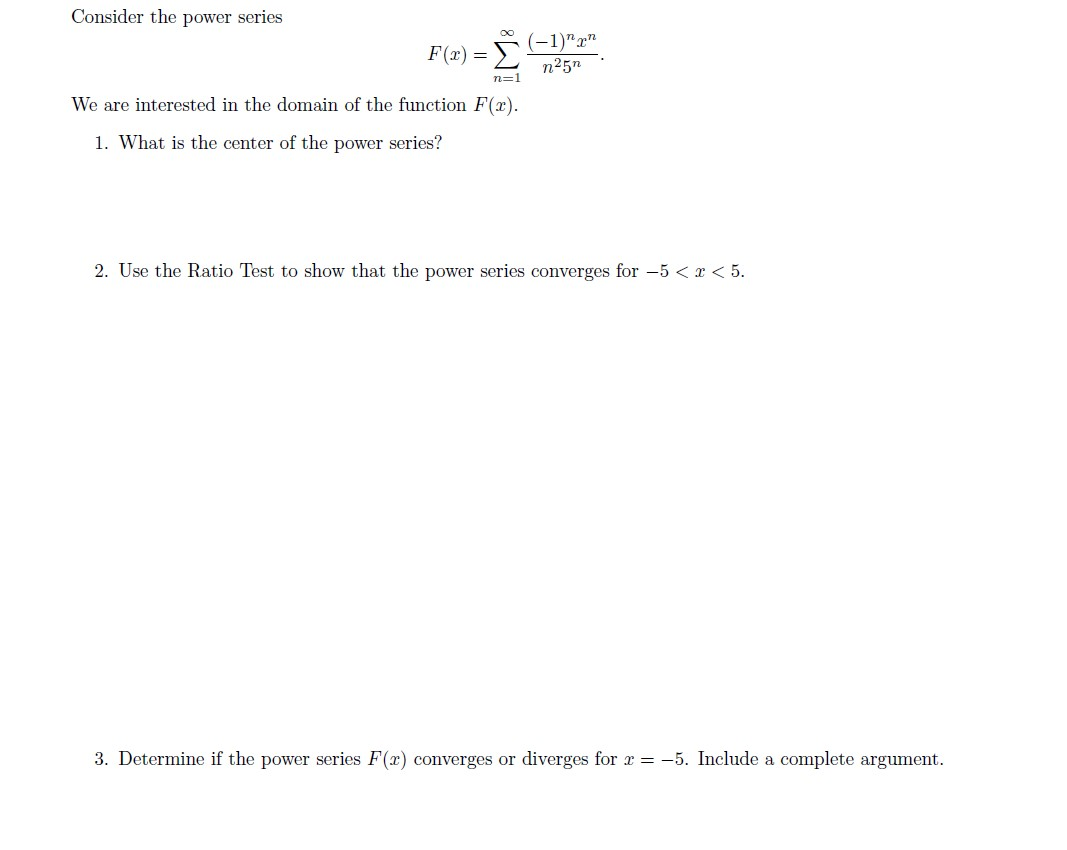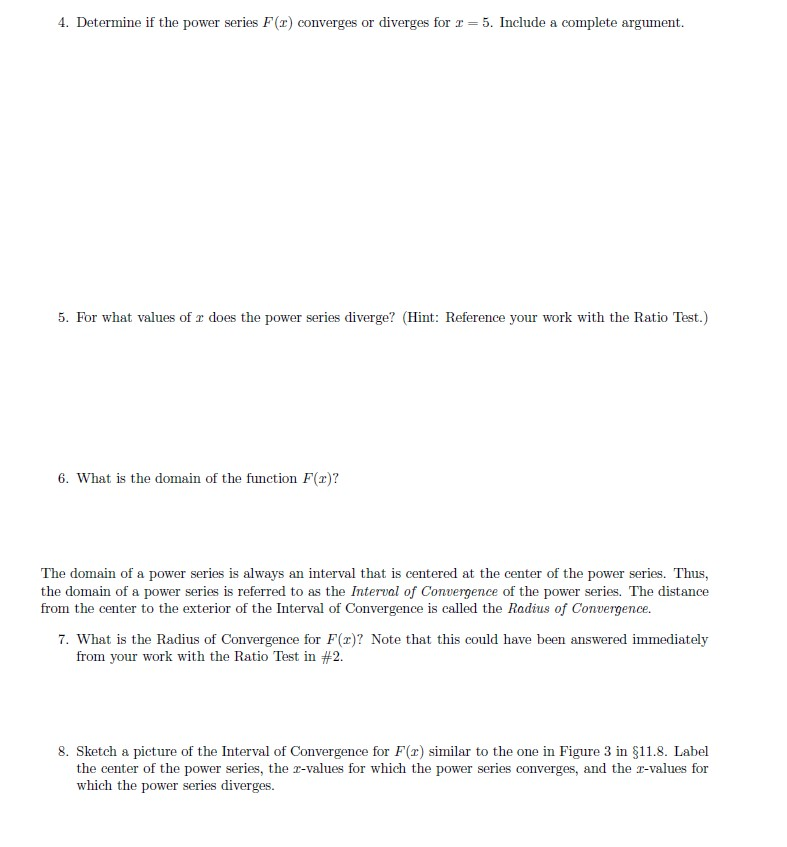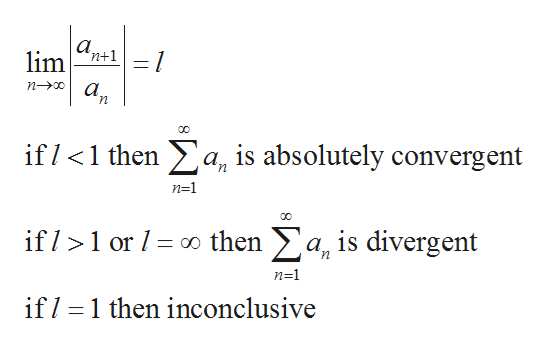# Consider the power series(-1)nΣF(x) =n25nn=1We are interested in the domain of the function F(x)1. What is the center of the power series?2. Use the Ratio Test to show that the power series converges for -5< x < 53. Determine if the power series F(r) converges or diverges for r-5. Include a complete argument 4. Determine if the power series F(r) converges or diverges for r5. Include a complete argument5. For what values of r does the power series diverge? (Hint: Reference your work with the Ratio Test.)6. What is the domain of the function F(x)?The domain of a power series is always an interval that is centered at the center of the power series. Thus,the domain of a power series is referred to as the Interval of Convergence of the power series. The distancefrom the center to the exterior of the Interval of Convergence is called the Radius of Convergence.7. What is the Radius of Convergence for F(r)? Note that this could have been answered immediatelyfrom your work with the Ratio Test in #2.8. Sketch a pieture of the Interval of Convergence for F(r) similar to the one in Figure 3 in §11.8. Labelthe center of the power series, ther-values for which the power series converges, and the r-values forwhich the power series diverges

Question
6 viewshelp_outlineImage TranscriptioncloseConsider the power series (-1)n Σ F(x) = n25n n=1 We are interested in the domain of the function F(x) 1. What is the center of the power series? 2. Use the Ratio Test to show that the power series converges for -5< x < 5 3. Determine if the power series F(r) converges or diverges for r-5. Include a complete argument fullscreenhelp_outlineImage Transcriptionclose4. Determine if the power series F(r) converges or diverges for r 5. Include a complete argument 5. For what values of r does the power series diverge? (Hint: Reference your work with the Ratio Test.) 6. What is the domain of the function F(x)? The domain of a power series is always an interval that is centered at the center of the power series. Thus, the domain of a power series is referred to as the Interval of Convergence of the power series. The distance from the center to the exterior of the Interval of Convergence is called the Radius of Convergence. 7. What is the Radius of Convergence for F(r)? Note that this could have been answered immediately from your work with the Ratio Test in #2. 8. Sketch a pieture of the Interval of Convergence for F(r) similar to the one in Figure 3 in §11.8. Label the center of the power series, ther-values for which the power series converges, and the r-values for which the power series diverges fullscreen
check_circle

Step 1

Hello. Since your question has multiple sub-parts, we will solve first three sub-parts for you. If you want remaining sub-parts to be solved, then please resubmit the whole question and specify those sub-parts you want us to solve.

Consider the given power series:

Step 2

A power series in power of (x-x0) , where x0 is the center of power series.

Now, compare it with the given power series.

Step 3

Therefore, 0 is the center of the power series.

Now, apply the ratio t...help_outlineImage Transcriptionclosea. lim n+1 n-0 а, n 00 if<1 then a, is absolutely convergent n=1 if/ 1 or o then a, is divergent n=1 if 1 then inconclusive fullscreen

### Want to see the full answer?

See Solution

#### Want to see this answer and more?

Solutions are written by subject experts who are available 24/7. Questions are typically answered within 1 hour.*

See Solution
*Response times may vary by subject and question.
Tagged in

### Math